# 车轮表面宏观形貌取向对高速轮轨水润滑黏着系数的影响

1中国科学院大学工程科学学院,北京 100049
2中国科学院力学研究所先进制造工艺力学重点实验室,北京 100190

# INFLUENCE OF MACROSCOPIC TOPOGRAPHY ORIENTATIONS OF WHEELS ON ADHESION COEFFICIENT OF HIGH SPEED WHEEL/RAIL UNDER WATER LUBRICATION

Jiang Huazhen12, Wang Baoan12, Li Zhengyang2*, Cai Baochun2, Yang Bing2, Ren Zhiyuan2

1 School of Engineering Science, University of Chinese Academy of Sciences, Beijing 100049, China
2 Key Lab of Mechanics in Advanced Manufacturing, Institute of Mechanics, Chinese Academy of Sciences, Beijing 100190, China

Abstract

Abstract Though tyre and asphalt are low elastic modulus materials, water lubrication resulting from hydrodynamic action would present even the speed is low. For high elastic modulus of materials, such as wheel and rail, water lubrication would present when the speed is over 200 km/h, causing potential unsafety for train operations. Increasing surface roughness will improve the wheel/rail adhesion coefficient. However it is shown that the topography orientation also has great effect on adhesion coefficient when the value of surface roughness is nearly the same under mixed lubrication. In this paper, a numerical analysis based on unified Reynolds equation was adopted. The behavior of three patterns of roughness orientations on wheels, i.e. longitudinal, transverse and rhombus, with high speed up to 500km/h under mixed lubrication were analyzed. The simulation results were compared with the results of the average flow model and the existing experimental results. It is concluded that the adhesion coefficients of wheel/rail decreased with speed increasing, while the adhesion coefficient of rhombus pattern is greater than that of the transverse, and transverse pattern is greater than that of the longitudinal. The adhesion coefficient is mainly depended on the ratio of asperity contact pressure to the total pressure. When the ellipticity $k<1$ in wheel/rail point contact, the lateral flow effect could not be neglected, the results of average flow model will result in error.

Keywords： elasto-hydrodynamic lubrication ; surface topography ; adhesion coefficient ; wheels/rails

0

Jiang Huazhen, Wang Baoan, Li Zhengyang, Cai Baochun, Yang Bing, Ren Zhiyuan. INFLUENCE OF MACROSCOPIC TOPOGRAPHY ORIENTATIONS OF WHEELS ON ADHESION COEFFICIENT OF HIGH SPEED WHEEL/RAIL UNDER WATER LUBRICATION[J]. Chinese Journal of Theoretical and Applied Mechanics, 2018, 50(1): 157-166 https://doi.org/10.6052/0459-1879-17-129

## 1 分析模型

$∂∂xρh312η∂p∂x+∂∂yρh312η∂p∂y=u∂(ρh)∂x+∂(ρh)∂t$ (1)

$h(x,y,t)=h0(t)+x22Rx+y22Ry+δ1(x,y,t)+δ2(x,y,t)+v(x,y,t)$ (2)

$v(x,y,t)=2E’\int \int_{\Gamma} p_{1}(x’,y’)+p_{s}(x’,y’) \sqrt{(x-x’)^{2}+(y-y’)^{2} }dx’dy’$ (3)

$u∂h∂x+∂h∂t=0$ (4)

$∂h∂x=0$(5)

$η=η0+η1p+η2p2+η3p3+η4p4$ (6)

$ρ=ρ0(1+0.6×10-9p1+1.7×10-9p)$ (7)

$p=Ps+Pl=∫‍∫Ω‍ps(x,y)dxdy+∫‍∫Ω‍pl(x,y)dxdy$ (8)

$μ=μsPs+μlPlp$ (9)

$γ̇=τη$

## 2 数值模拟

$z(x,y)=\begin{equation} \left \{ \begin{array} \ \sqrt{R^2-x_{s}^{2}-y_{s}^{2}}-Z_{a}, \sqrt{x_{s}^{2}+y_{s}^{2}} \le R_{1} \\ A(\sqrt{x_{s}^{2}+y_{s}^{2}}-R_{1})(\sqrt{x_{s}^{2}+y_{s}^{2}}-R_{2}) \\ R_{1} < \sqrt{x_{s}^{2}+y_{s}^{2}} \le R_{2} \\ rand(z), else \end{array} \right. \end{equation}$ (11)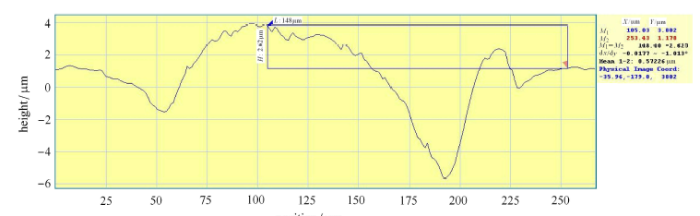Fig. 1   The real cross-section profile of a single laser pattern. The diameter of the pattern is about 150 m, the height over the surface is about 2.5 m

$xs=x-2800n,ys=y-2800n,rhombuspatternxs=x-2800n,ys=y-1400n,transversepattern(nisthenumberofiterations)xs=x-1400n,ys=y-2800n,longitudinalpattern$ (12)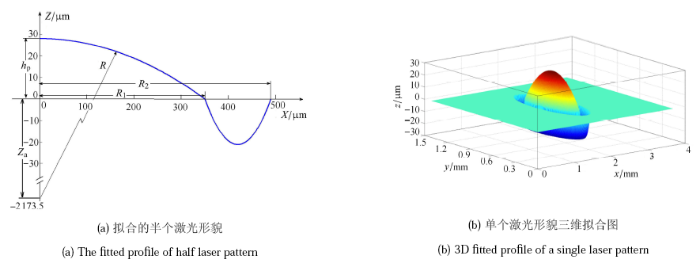Fig. 2   The fitted profiles of laser pattern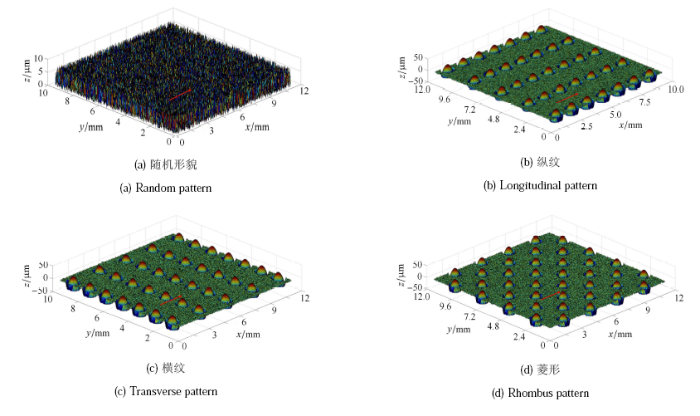Fig. 3   The reconstructed surface for calculation. The arrows indicate the direction of rolling

Table 1   The parameters used in the numerical simulation

Parameters
(wheels/rails or water)
Value
Effective elastic modulus
E'/ GPa
242
Poisson's ratio $\nu$0.3
$R_{1x}$/m0.45
$R_{2y}$/m0.30
Temperature/${^\circ}$C20
Normal load/N8.50#x00D7;10$4$
Velocity/(km$\cdot$h$^{-1}$)100, 200, 300, 400, 500
Creep rate %0.0001,0.30, 0.50, 1.0, 1.5, 2.0
Viscosity at room
temperature} $\eta _{0}/(\Pa}\cdot s)$
0.001 01
Density of water at room
temperature $\rho _{0}/(g\cdot cm^{-3}$
1.00

## 3 结果与讨论

### 3.1 压力与膜厚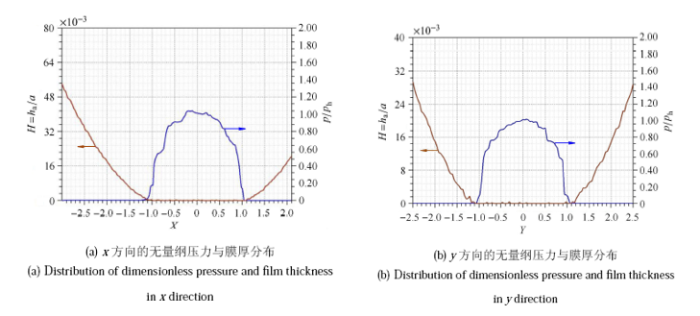Fig. 4-1   Distribution of dimensionless pressure and film thickness and dimensionless film thickness contour with random topography(a) Distribution of dimensionless pressure and film thickness in $x$ direction (b) Distribution of dimensionless pressure and film thickness in $y$ direction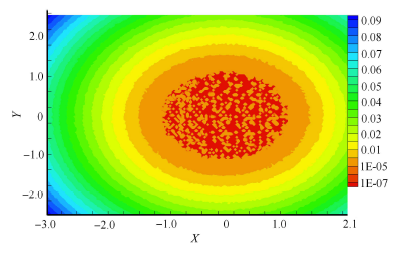Fig. 4-2   Distribution of dimensionless pressure and film thickness and dimensionless film thickness contour with random topography(c) Contour plot of film thickness $H=h_{\rm a}/a$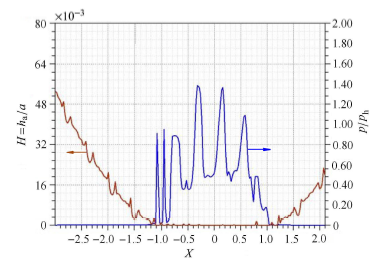Fig. 5-1   Distribution of dimensionless pressure and film thickness and dimensionless film thickness contour with transverse pattern (a) Distribution of dimensionless pressure and film thickness In x direction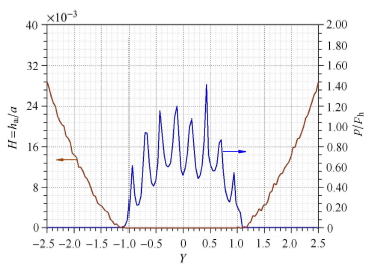Fig. 5-2   Distribution of dimensionless pressure and film thickness and dimensionless film thickness contour with transverse pattern (b) Distribution of dimensionless pressure and film thickness in y direction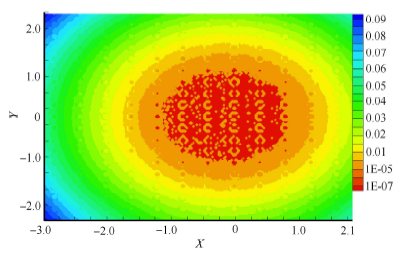Fig. 5-3   Distribution of dimensionless pressure and film thickness and dimensionless film thickness contour with transverse pattern (c) Plot of film thickness $H=h_{\rm a}/a$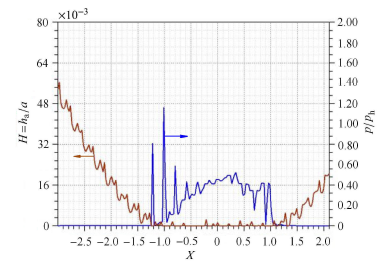Fig. 6-1   Distribution of dimensionless pressure and film thickness and dimensionless film thickness contour with longitudinal pattern (a) Distribution of dimensionless pressure and film thickness in $x$ direction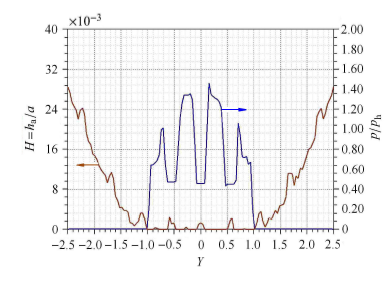Fig. 6-2   Distribution of dimensionless pressure and film thickness and dimensionless film thickness contour with longitudinal pattern (b) Distribution of dimensionless pressure and film thickness in $y$ direction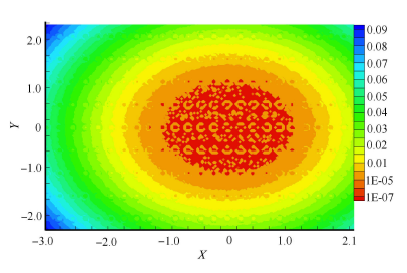Fig. 6-3   Distribution of dimensionless pressure and film thickness and dimensionless film thickness contour with longitudinal pattern (c) Contour plot of film thickness $H=h_{\rm a}/a$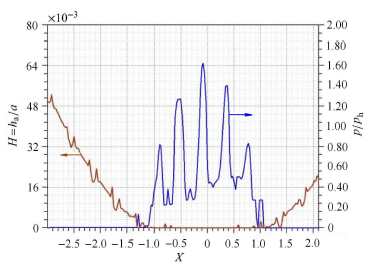Fig. 7-1   Distribution of dimensionless pressure and film thickness and dimensionless film thickness contour with rhombus pattern (a) Distribution of dimensionless pressure and film thickness in $x$ direction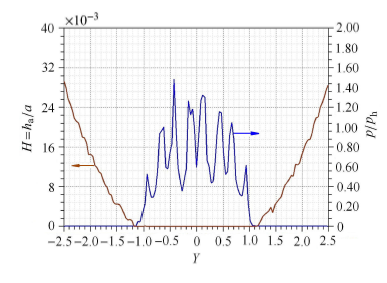Fig. 7-2   Distribution of dimensionless pressure and film thickness and dimensionless film thickness contour with rhombus pattern (b) Distribution of dimensionless pressure and film thickness in $y$ direction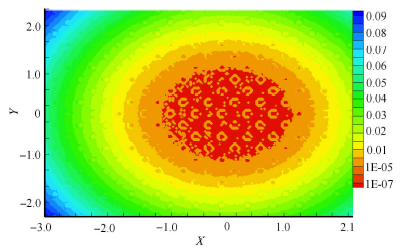## 4 结论

(1) 当蠕滑率一定并且运动速度超过200 km/h,三种规则形貌的轮轨黏着系数都随速度的增大而降低,其中菱形的黏着系数大于 横纹的黏着系数,横纹的黏着系数又大于纵纹的黏着系数.

(2) 在混合润滑条件下,平均流量模型只对某些形状的接触区适用,而统一雷诺方程模型可适用于任何形状的接触区,并且计算 结果物理意义明确、清晰.

(3) 影响黏着系数的主要原因是固体接触压力与总压力之比.

The authors have declared that no competing interests exist.

## 参考文献 原文顺序 文献年度倒序 文中引用次数倒序 被引期刊影响因子〈〉